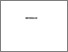# Pulmonary oxygen uptake kinetics in middle-and long-distance runners.

KILDING, Andrew E. (2003). Pulmonary oxygen uptake kinetics in middle-and long-distance runners. Doctoral, Sheffield Hallam University (United Kingdom)..Preview
PDF (Version of Record)
10702837.pdf - Accepted Version

## Abstract

The overall aim of this thesis was to evaluate the importance of pulmonary oxygen uptake (VO) kinetics, in the moderate-domain, in the assessment of endurance-trained runners. Accordingly, there were five objectives: 1) to quantify the reproducibility of measures of VO kinetics; 2) to characterise and compare VO kinetics during the on-and off-transients in middle-distance (MD) and long-distance (LD) runners; 3) to assess the relationship between VO kinetics and maximal VO (VO[2Max]), ventilatory threshold (V[T]) and running economy (RE); 4) to determine the relationship between VO kinetics and running performance and 5) to assess whether VO kinetics is a determinant of running performance.Twelve participants performed two assessments of VO kinetics on separate days to determine the reproducibility. Paired t-tests showed that parameters from test 1 and test 2 did not differ (P >0.05). Furthermore, narrow 95% limits of agreement (LOA), low measurement and method error suggested that the on- and off-transient time-constants (pion and pioff), mean response times (MRT[on] and MRT[off]) and amplitudes (A[on] and A[off]) were reproducible and could be used for the assessment of runners. Subsequently, VO kinetics were compared in 10 MD and 10 LD runners. There was a tendency for pion (12.5 +/- 2.3 s vs. 14.2 +/- 3.1 s, P = 0.178) and pioff (24.1 +/- 2.3 s vs. 27.1 +/- 3.0 s, P = 0.023) to be shorter in LD than MD runners respectively, despite similar VO[2Max] (MD = 60.0 +/- 4.9 ml-kg[-1]min[-1]; LD = 59.0 +/- 6.3 ml-kg[-1]-min[-1], P = 0.689). Differences in VO kinetics between MD and LD runners were attributed to approaches to training since the volume of training was greater in LD (64.0 +/- 15.7 km-wk[-1]) than MD (47.5 +/- 15.7 km-wk[-1]) runners (P = 0.047). To detail the relationships between VO kinetics and other measures of aerobic function (VO[2max], V[t] and RE), 16 MD and 16 LD runners were assessed. Relationships existed between pion and VO[2max] (r = -0.72, P = 0.002), V[t] (r = -0.66, P = 0.006) and RE (r = -0.59, P = 0.016) in LD runners, but not in MD runners (P >0.05). In addition, pion was related to the volume of training in MD (r = -0.63 , P = 0.009) and LD runners (r = -0.65, P = 0.006).The importance of VO kinetics for 5 km running performance was investigated in 36 endurance trained runners. Runners were categorised as high n=10), low (n=10) and combined [MD + LD (n=36)] performers according to running ability after performing a self-paced 5 km time-trial. Mean (+/-SD) speed for the 5 km time-trial was 5.2 +/-1.0 m-s[-1] (high), 4.5 +/- 0.2 m-s[-1] (low) and 4.9 +/- 0.3 m-s[-1] (combined). Measures of on- and off- transient VO kinetics, VO[2max], V[t] and RE were also determined. Data were explored using bi-variate correlations, ANCOVA and multiple regression techniques. In high and low performers, V0 kinetic parameters were not related to running performance. In combined runners, pion, pioff, MRT[on] and MRT[off] were related (r = -0.54, P = 0.001; r = -0.36, P = 0.030; r = -0.50, P = 0.002; r = -0.63, P = 0.003) to running performance. Stepwise multiple regression models were used to identify the primary determinant(s) of 5 km running performance for each group. In high performers, VO[2Max] and RE were included in the model (r = 0.92, R = 0.85, SEE = 0.08 m-s[-1]; SEE% = 1.5). In low performers, VO[2Max] was included in the model (r = 0.76, R = 0.57, SEE = 0.15 m-s[-1], SEE% = 3.3). In combined runners, V0[2Max] RE and MR[off] were included in the model (r = 0.87, R-2 = 0.75, SEE = 0.17 m-s[-1], SEE% = 3.5).Collectively, the results suggest that: 1) VO kinetics can be reproducibly determined using a single visit protocol; 2) measures of VO kinetics are sensitive enough to differentiate MD and LD runners; 3) relationships between VO kinetics and other measures of aerobic function exist in LD runners, but not in MD runners; 4) VO kinetics differ between high and low performers, but do not relate to running performance and 5) VO kinetics discriminate between high and low performers but only contribute minimally to the prediction of running performance in a multiple regression model for combined MD and LD runners.

Item Type: Thesis (Doctoral) Thesis advisor - Fysh, Mary Thesis (Ph.D.)--Sheffield Hallam University (United Kingdom), 2003. Sheffield Hallam Doctoral Theses EPrints Services 10 Apr 2018 17:23 26 Apr 2021 13:26 https://shura.shu.ac.uk/id/eprint/20739View Item